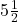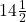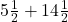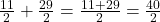## Write an equation to represent the problem, be sure to explain the meaning of any variables you use, Jaden dog was 5 and a half inches tall

Question

Write an equation to represent the problem, be sure to explain the meaning of any variables you use, Jaden dog was 5 and a half inches tall when it was a puppy, now her dog is 14 and a half inches taller than that, how tall is the dog now​

in progress 0
4 weeks 2021-08-18T10:52:13+00:00 1 Answers 2 views 0

The dog is 20 inches tall now.

Step-by-step explanation:

Given that:

Height of dog when it was a puppy =inches

Height of dos now =than previous height

It means that we will add this height in the height of dog when it was a puppy.

Height of dog now =Height of dog =Height of dog = 20 inches

Hence,

The dog is 20 inches tall now.